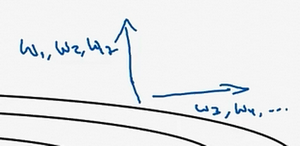## 第二周：优化算法 (Optimization algorithms)

### 2.1 Mini-batch 梯度下降（Mini-batch gradient descent）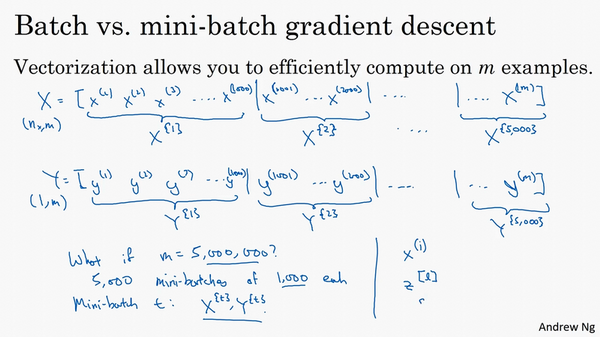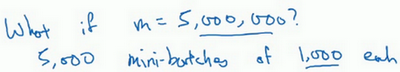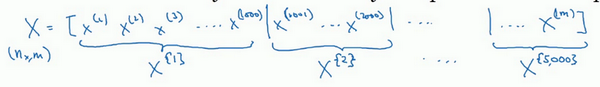$Y$也要进行相同处理，你也要相应地拆分$Y$的训练集，所以这是$Y^{\{1\}}$，然后从$y^{(1001)}$$y^{(2000)}$，这个叫$Y^{\{2\}}$，一直到$Y^{\{ 5000\}}$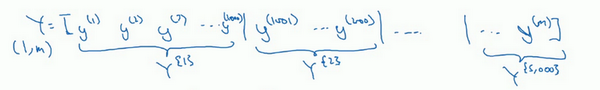mini-batch的数量$t$组成了$X^{\{ t\}}$$Y^{\{t\}}$，这就是1000个训练样本，包含相应的输入输出对。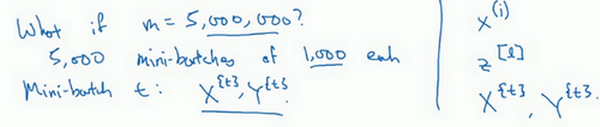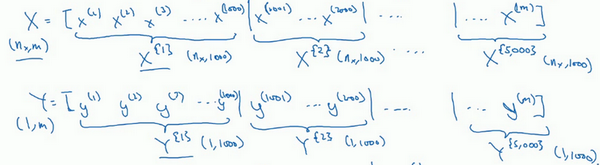$X^{\{ t\}}$$Y^{\{ t\}}$的维数：如果$X^{\{1\}}$是一个有1000个样本的训练集，或者说是1000个样本的$x$值，所以维数应该是$(n_{x},1000)$$X^{\{2\}}$的维数应该是$(n_{x},1000)$，以此类推。因此所有的子集维数都是$(n_{x},1000)$，而这些（$Y^{\{ t\}}$）的维数都是$(1,1000)$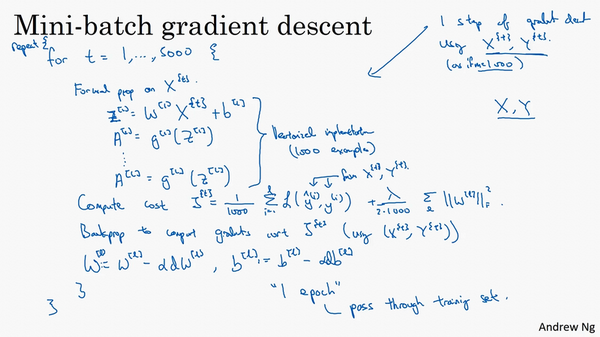### 2.2 理解mini-batch梯度下降法（Understanding mini-batch gradient descent）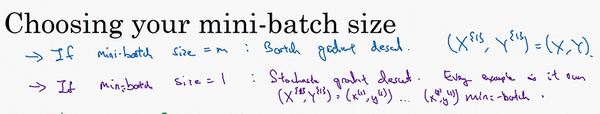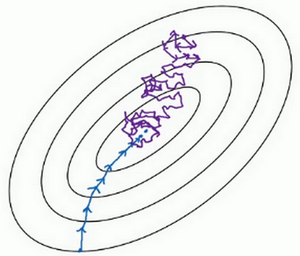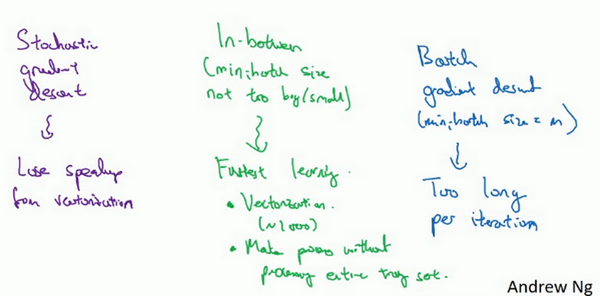mini-batch梯度下降法，我们从这里开始，一次迭代这样做，两次，三次，四次，它不会总朝向最小值靠近，但它比随机梯度下降要更持续地靠近最小值的方向，它也不一定在很小的范围内收敛或者波动，如果出现这个问题，可以慢慢减少学习率，我们在下个视频会讲到学习率衰减，也就是如何减小学习率。

### 2.3 指数加权平均数（Exponentially weighted averages）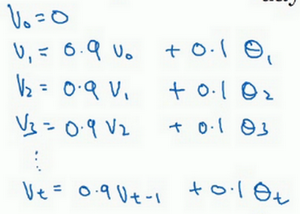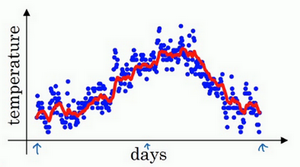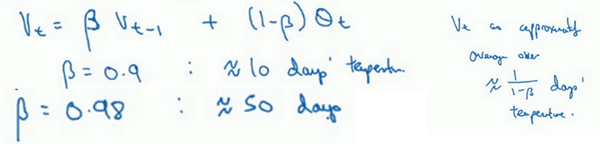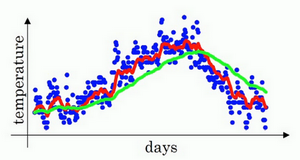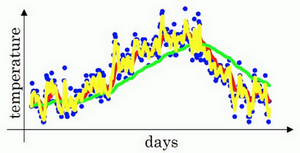### 2.4 理解指数加权平均数（Understanding exponentially weighted averages）

${{v}_{t}}=\beta {{v}_{t-1}}+(1-\beta ){{\theta }_{t}}$

$\beta=0.9$的时候，得到的结果是红线，如果它更接近于1，比如0.98，结果就是绿线，如果$\beta$小一点，如果是0.5，结果就是黄线。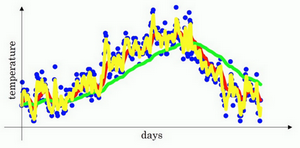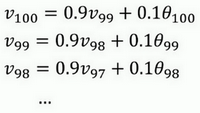$v_{100} = 0.1\theta_{100} + 0.9(0.1\theta_{99} + 0.9v_{98})$

$v_{100} = 0.1\theta_{100} + 0.9(0.1\theta_{99} + 0.9(0.1\theta_{98} +0.9v_{97}))$

$v_{100} = 0.1\theta_{100} + 0.1 \times 0.9 \theta_{99} + 0.1 \times {(0.9)}^{2}\theta_{98} + 0.1 \times {(0.9)}^{3}\theta_{97} + 0.1 \times {(0.9)}^{4}\theta_{96} + \ldots$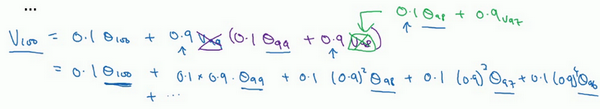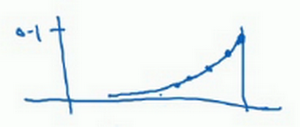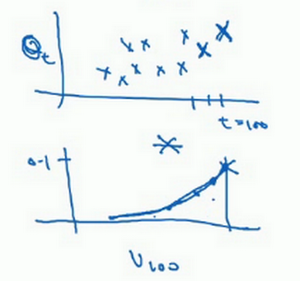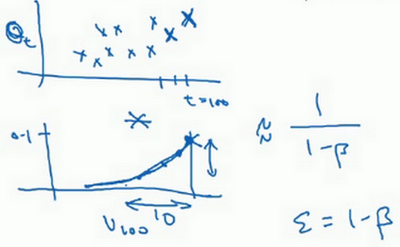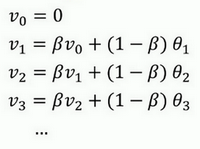### 2.5 指数加权平均的偏差修正（Bias correction in exponentially weighted averages）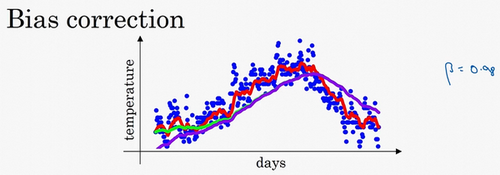${{v}_{t}}=\beta {{v}_{t-1}}+(1-\beta ){{\theta }_{t}}$

$v_{2} = 0.98v_{1} + 0.02\theta_{2}$，如果代入$v_{1}$，然后相乘，所以$v_{2}= 0.98 \times 0.02\theta_{1} + 0.02\theta_{2} = 0.0196\theta_{1} +0.02\theta_{2}$，假设$\theta_{1}$$\theta_{2}$都是正数，计算后$v_{2}$要远小于$\theta_{1}$$\theta_{2}$，所以$v_{2}$不能很好估测出这一年前两天的温度。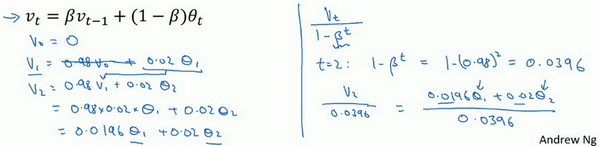### 2.6 动量梯度下降法（Gradient descent with Momentum）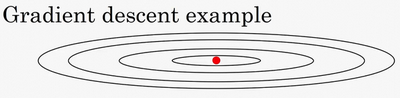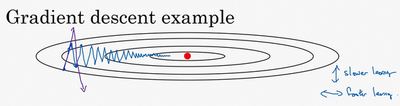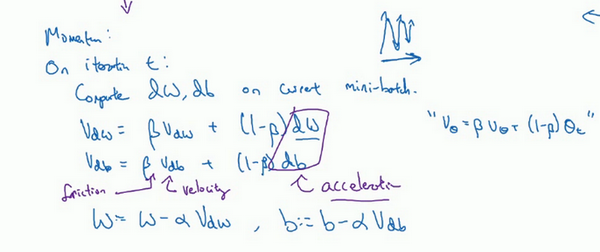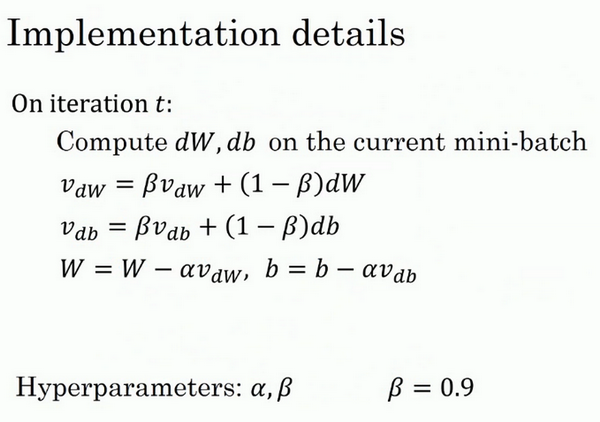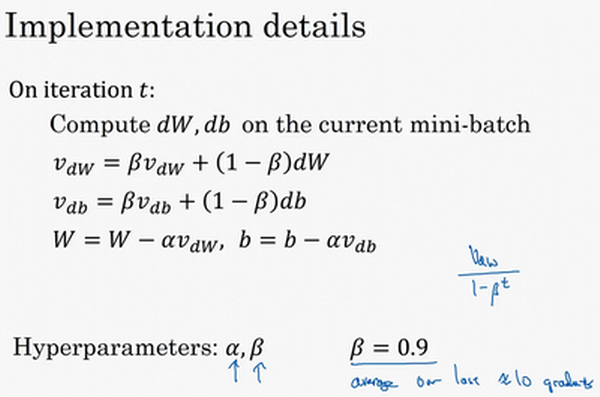### 2.7 RMSprop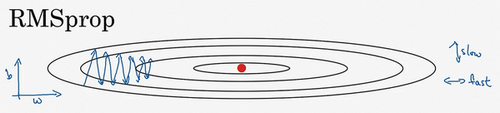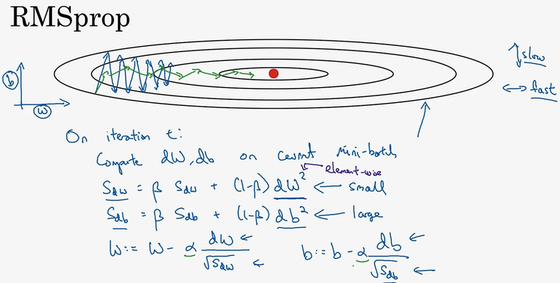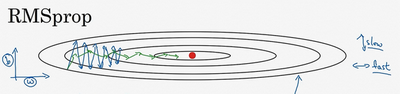RMSprop的影响就是你的更新最后会变成这样（绿色线），纵轴方向上摆动较小，而横轴方向继续推进。还有个影响就是，你可以用一个更大学习率$a$，然后加快学习，而无须在纵轴上垂直方向偏离。